# Chapter 27, Problem 050 In the figure R1 = 2.390, the ammeter resistance is zero, and...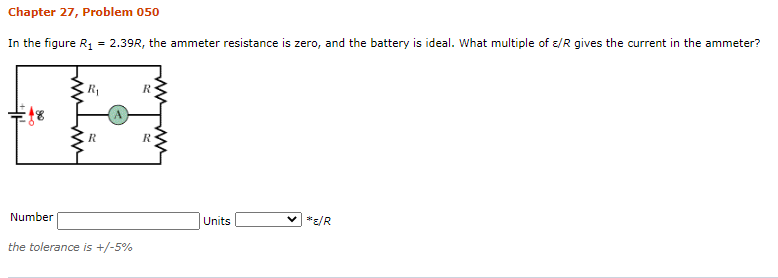Chapter 27, Problem 050 In the figure R1 = 2.390, the ammeter resistance is zero, and the battery is ideal. What multiple of E/R gives the current in the ammeter? R E1% R R Number Units VER the tolerance is +/-5%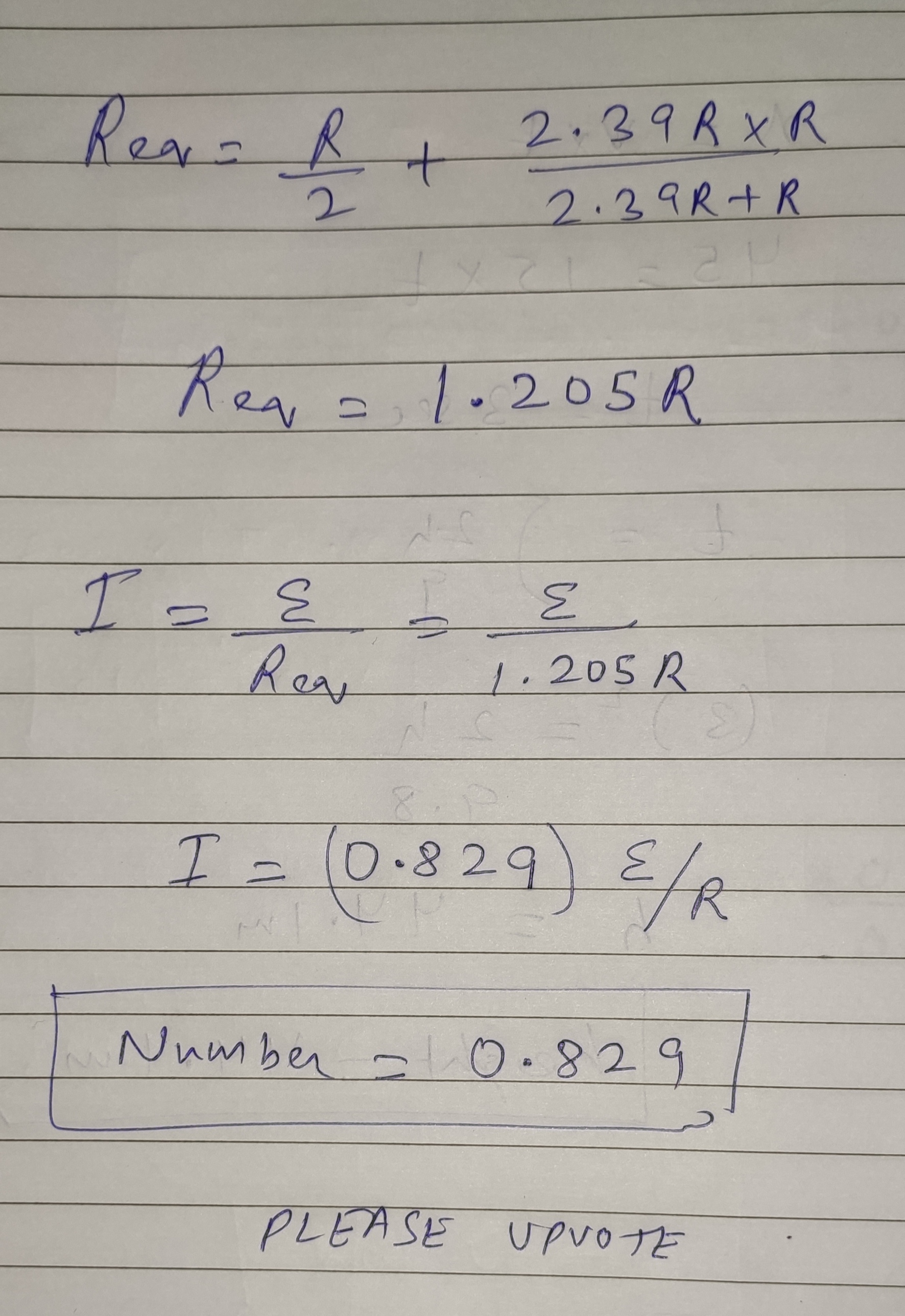#### Earn Coin

Coins can be redeemed for fabulous gifts.

Similar Homework Help Questions
• ### Chapter 27, Problem 050 In the figure R1 = 1.39R, the ammeter resistance is zero, and...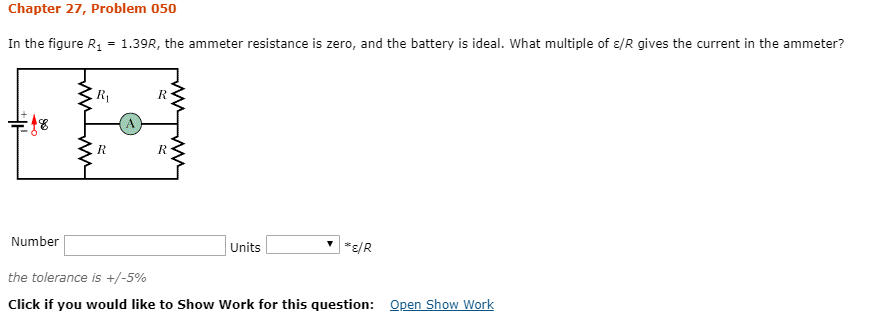Chapter 27, Problem 050 In the figure R1 = 1.39R, the ammeter resistance is zero, and the battery is ideal. what multiple of ε/R gives the current in the amnmeter? Number Units the tolerance is +/-5% Click if you would like to Show Work for this question: Open Show Work

• ### In the figure R1 = 2.83R, the ammeter resistance is zero, and the battery is ideal....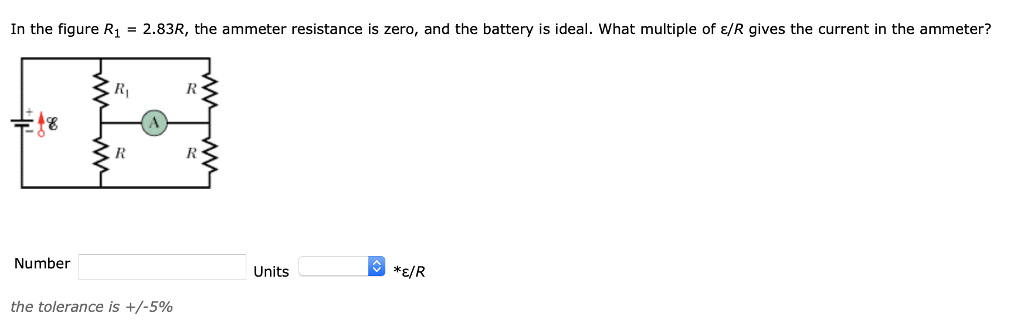In the figure R1 = 2.83R, the ammeter resistance is zero, and the battery is ideal. what multiple of ε/R gives the current in the ammeter? Number Units *E/R the tolerance is +/-590

• ### Current in ammeter (will rate lifesaver!)In Fig. 27-57, R1 = 3.77R, the ammeter resistance is zero, and the battery is ideal. What multiple of ε/R gives the current in the ammeter?Figure 27-57

• ### Chapter 27, Problem 044 In the figure R1 = 1302, R2 = R3 = 44.0 2,...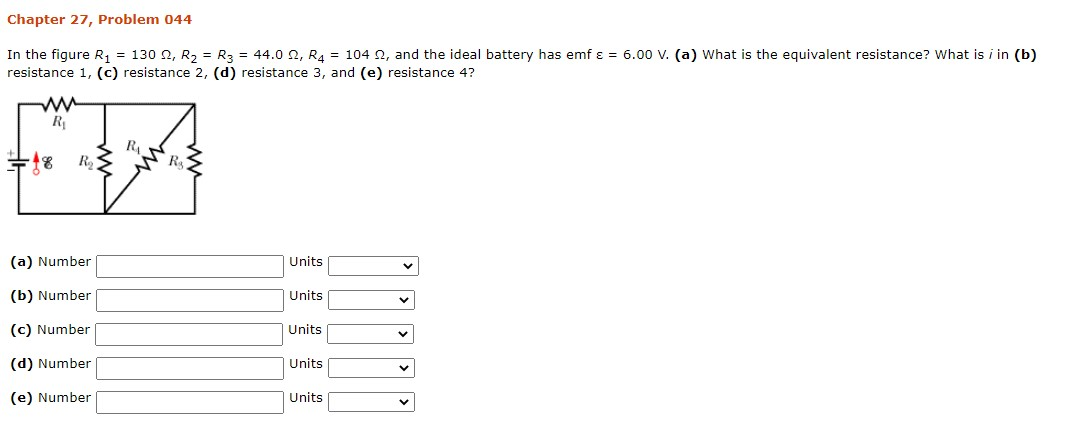Chapter 27, Problem 044 In the figure R1 = 1302, R2 = R3 = 44.0 2, R4 = 1042, and the ideal battery has emf ε = 6.00 V. (a) What is the equivalent resistance? What is i in (b) resistance 1, (c) resistance 2, (d) resistance 3, and (e) resistance 4? R RA RA (a) Number Units (b) Number Units (c) Number Units V (d) Number Units (e) Number Units

• ### Chapter 27, Problem 029 In the figure R1 6.87 Ω, R2" 20.6 Ω, and the ideal...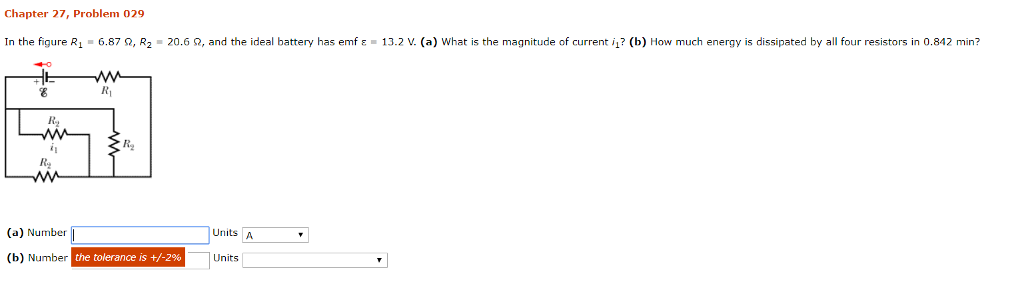Chapter 27, Problem 029 In the figure R1 6.87 Ω, R2" 20.6 Ω, and the ideal battery has emf ε·13.2 V. (a) what is the magnitude of current i? (b) How much energy is dissipated by all four resistors in 0.842 min? R2 R2 Rg (a) Number「 (b) Number Units A the tolerance is +/-2% Units

• ### Chapter 27, Problem 044 GO In the figure R1 = 83.0 2, R2 = R3 =...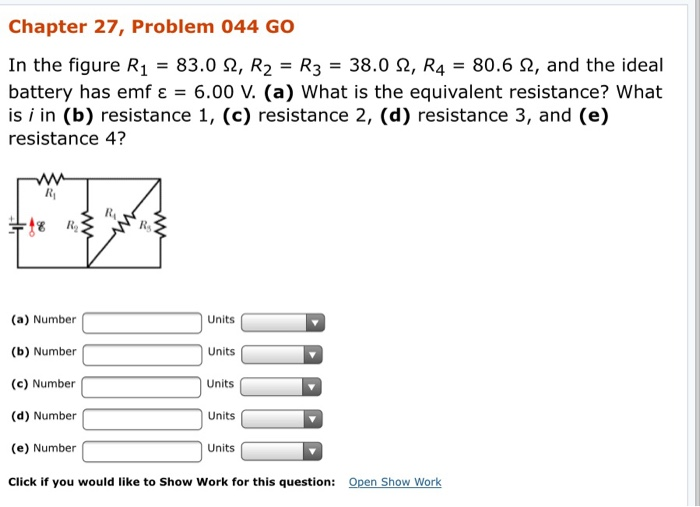Chapter 27, Problem 044 GO In the figure R1 = 83.0 2, R2 = R3 = 38.0 2, R4 = 80. 62, and the ideal battery has emf ε = 6.00 V. (a) What is the equivalent resistance? What is i in (b) resistance 1, (c) resistance 2, (d) resistance 3, and (e) resistance 4? (a) Number (b) Number (c) Number Units Units Units units Units (d) Number (e) Number Click if you would like to Show Work for this...

• ### ES Chapter 27, Problem 033 GO In the figure the current in resistance 6 is 16...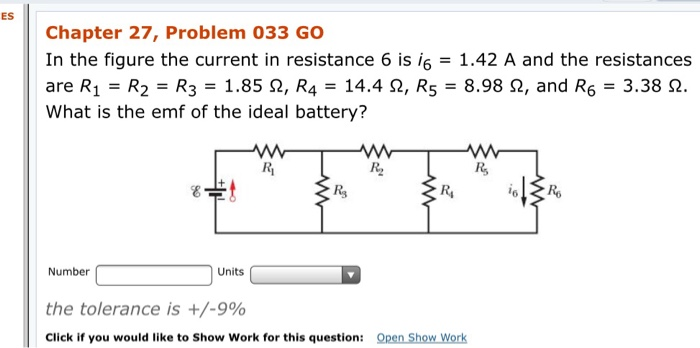ES Chapter 27, Problem 033 GO In the figure the current in resistance 6 is 16 = 1.42 A and the resistances are Ri = R2 = R3 = 1.85 92, R4 = 14.4 S2, R5 = 8.98 2, and Ro = 3.38 2. What is the emf of the ideal battery? 8 = Number Units the tolerance is +/-9% Click if you would like to Show Work for this question: Open Show Work

• ### Chapter 27, Problem 044 GO In the figure R1 = 120 12, R2 = R3 =...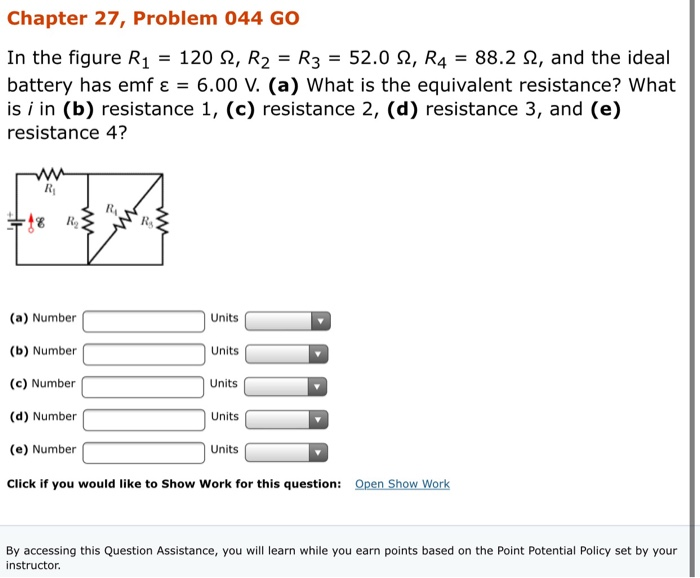Chapter 27, Problem 044 GO In the figure R1 = 120 12, R2 = R3 = 52.0 2, R4 = 88.2 12, and the ideal battery has emf ε = 6.00 V. (a) What is the equivalent resistance? What is i in (b) resistance 1, (c) resistance 2, (d) resistance 3, and (e) resistance 4? (a) Number Units (b) Number Units (c) Number (d) Number Units Units Units C (e) Number Click if you would like to Show Work for...

• ### Chapter 27, Problem 029 In the figure R1 = 5.65 2, R2 = 17.0 2, and...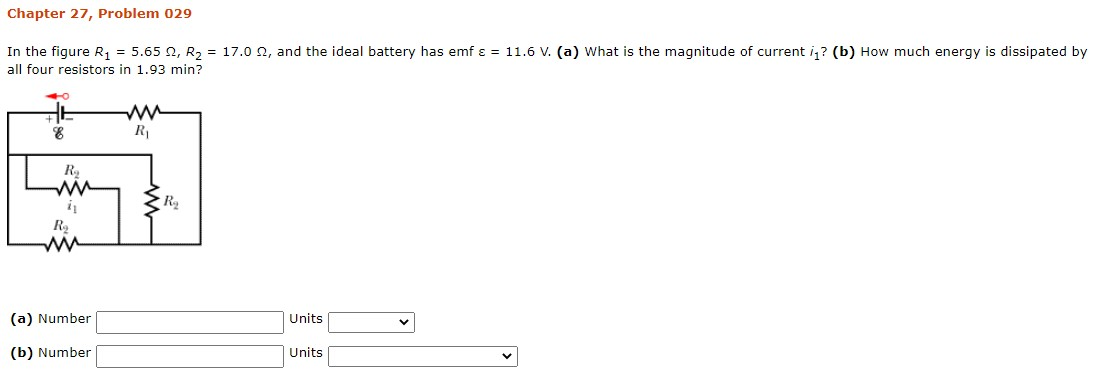Chapter 27, Problem 029 In the figure R1 = 5.65 2, R2 = 17.0 2, and the ideal battery has emf = = 11.6 V. (a) What is the magnitude of current i? (b) How much energy is dissipated by all four resistors in 1.93 min? 8 RI RY RO wu (a) Number Units (b) Number Units

• ### Chapter 27, Problem 041 In the figure £1 = 3.05 V, €2 = 0.889 V, R1...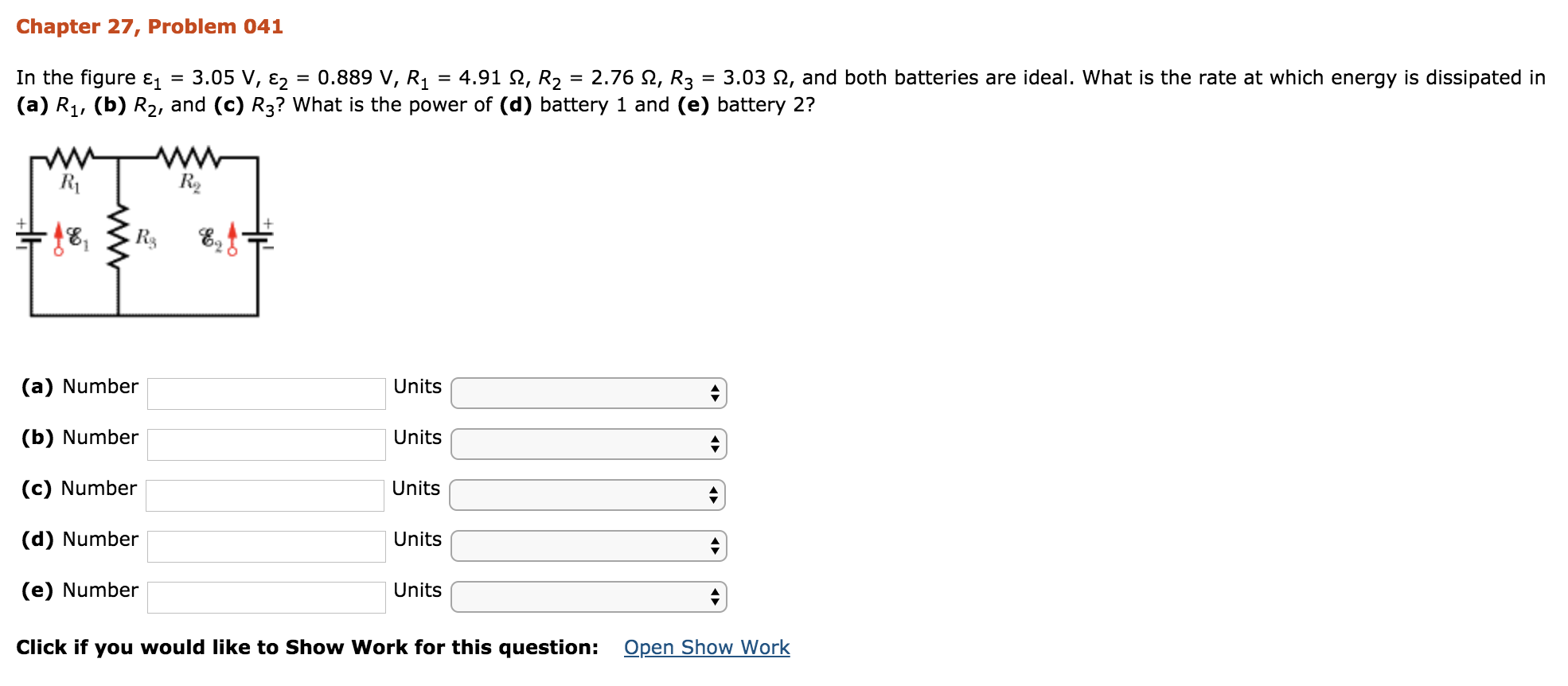Chapter 27, Problem 041 In the figure £1 = 3.05 V, €2 = 0.889 V, R1 = 4.91 12, R2 = 2.76 12, R3 = 3.03 12, and both batteries are ideal. What is the rate at which energy is dissipated in (a) R4, (b) R2, and (c) R3? What is the power of (d) battery 1 and (e) battery 2? 18, R. & 1 - (a) Number Units (b) Number Units (c) Number Units (d) Number Units (e) Number...